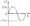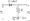# Third Semester Electronic Circuits July 2006

Note : 1. Answer any FIVE full questions.

1 a. Analyse the clipper circuit of Fig 1(a) and draw its output waveform and transfer characteristic curve.Fig 1 (a).

b.  Draw the circuit of a Ml wave bridge rectifier with capacitor filter and explain its operation. Derive an expression for its ripple factor.

c.  Explain the operation of a full wave voltage doubler circuita.

2 a. Discuss the causes for bias instability in a transistor.

b.  Explain how a diode can be used in a transistor circuit to compensate for changes in Vbe-

c.  In the circuit of Fig 2(c) shown below, Vcc – 24V, Rc = lOKQRe = 2700. If a silicon transistor is used with (B = 45 and if under quiescent conditions Vce = 5V, determine3   a; Draw the hybrid small signal model of a transistor and explain the significance of each component in the model.

b.  Using h-parameter model for a transistor in C.E configuration, Derive expressions for A], Z„ Av and Y0 of the amplifier.

c.  In the circuit of Fig 3(c) shown below, Calculate the input impedance Rj. The transistor parameters are hie = 1100H, hre = 2.5 x 10′4, hoe = 25ja A/V.

4 a. State and prove Miller’s theorem.

b. Derive expressions for transistor input conductance gbe and output conductance gce.

c. Discuss different types of distortions in amplifiers.

5 a. Draw a feedback amplifier in block diagram form. Identify each block and explain their function.

b.  Derive expressions for input and output resistances of a current shunt feedback amplifier.

c.  An amplifier with an open loop voltage gain Av = 1000 ± 100 is available. It is necessary to have an amplifier whose voltage gain varies by no more than ± 0.1 percent Find:

i)      The reverse transmission factor p of the F.B network and

ii) The gain with F.B.

6 a.   Discuss different types of power amplifiers in terms of their efficiency and applications.

b.  Draw the circuit diagram of a class B push pull amplifier and explain the operation with relevant wave forms. Also derive the maximum conversion efficiency of this amplifier.

c.  Calculate the peak power dissipation in each transistor and the maximum power output in a class B push pull amplifier it Vcc” 10V and =4Q.

7 a. Draw the circuit and explain how to measure the differential input resistance of an op. Amp.

b. Design an op. Amp Schmitt trigger circuit to meet the following specifications. = 4V, Vltp– -2 V, V0 = ± 12V.

c. Explain the working of a D/A converter with R and 2R resistors. Derive the expression for the ouput voltage.

8 a. Explain the working of an op. Amp positive clipper.

b. Draw and explain the working of a Sample and Hold circuit.

c.  List the important features of 555 timer. Explain how an a stable multivibrator using.555 timer can be used as a free- running ramp generator.